# Molality Formula

Molality Formula

Molality is a term used to describe the concentration of a solution. It is equal to the moles of solute (the substance being dissolved) divided by the kilograms of solvent (the substance used to dissolve). Molality is not as common as its counterpart molarity but it is used in very specific calculations, most notably involving colligative properties (boiling point elevation, freezing point depression).Molality Formula Questions:

1. What is the molality of a solution if 1.57 moles of solute are dissolved in 2.35 kilograms of water?

Answer: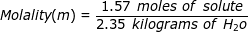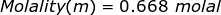2. What is the molality of a solution if 124.2 grams of NaOH are dissolved in 1.00 liter of water? (The density of water is equal to 1.00)

Answer: Water is referred to as the universal solvent and the density is typically given as 1.00 grams per liter. This makes for an easy conversion from liters to kilograms.

In this problem the 124.2 grams of NaOH will need to be converted to moles (for moles of solute) and one liter of water will need to be converted into kilograms (for kilograms of solvent) using the density.

First, find the molar mass of NaOH.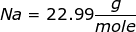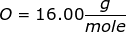NaOH = 22.99 + 16.00 + 1.01 = 40.00Second, convert the grams of NaOH into moles, using the molar mass.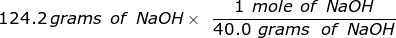= 3.105 moles of NaOH

Third, convert the liter of water into kilograms using the density.= 1.00 kilograms

Finally, use the molality equation to solve.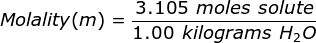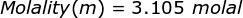Related Links: